### 1.化标准型

• 把不等式约束改成等式约束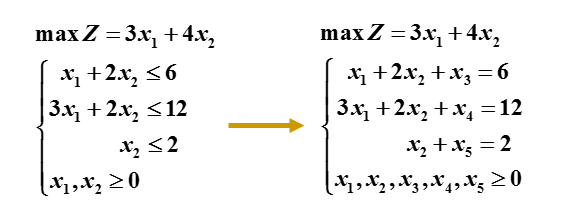### 2.单纯形表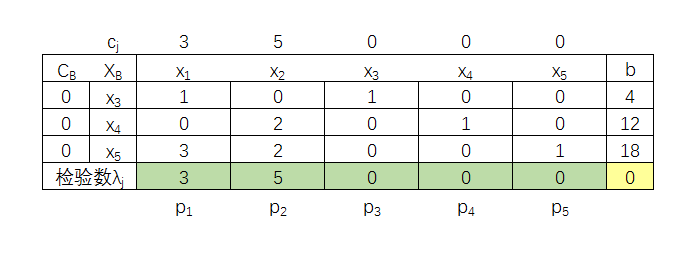cj xj 对应在目标函数中的系数
CB XB 对应在目标函数中的系数
pj 为 xjT
b： 为约束方程右边的值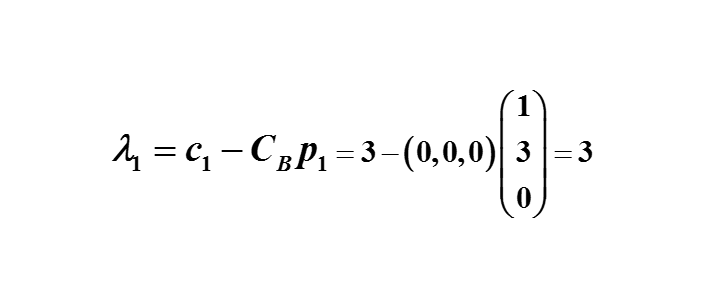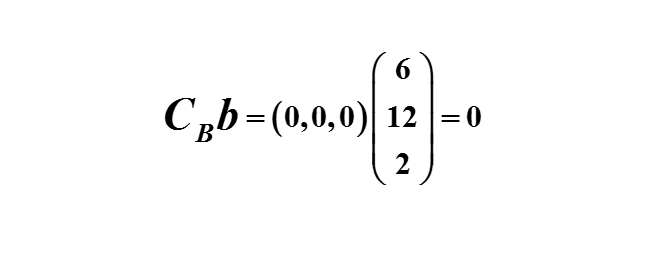### 3.迭代方法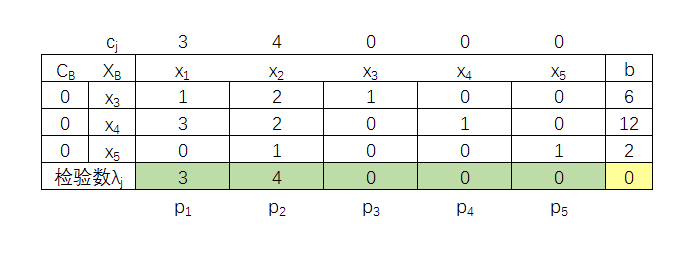• 确定进基变量

X=(x1,x2,x3,x4,x5)T
X0=(0,0,6,12,2)T→Z0=0
X1=(0,θ,x3‘,x4‘,x5’)T→Z1=4θ↑
maxZ=3x1+4x2=3×0+4×θ=4θ
X1=(θ,0,x3’‘,x4’‘,x5’’)T→Z1=3θ↑
maxZ=3x1+4x2=3×θ+4×0=3θ

λk=max{λjj>0}→xk为进基变量
λ2=max{λ12>0}→x2为进基变量

• 确定离基变量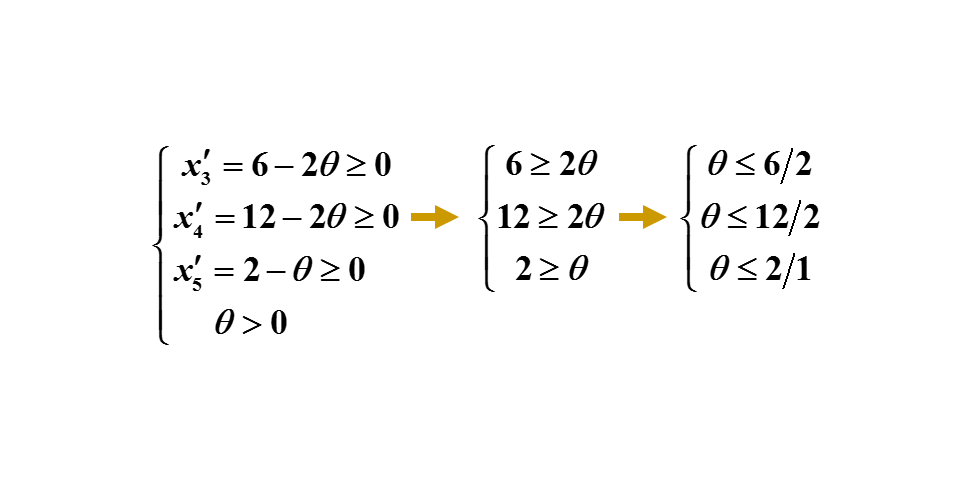θ=min{6/2,12/2,2/1}=2→x5为离基变量

x3‘=6-2θ=2
x4‘=12-2θ=8
x5‘=2-θ=0
X1=(0,2,2,8,0)T→Z1=8↑

• 换基运算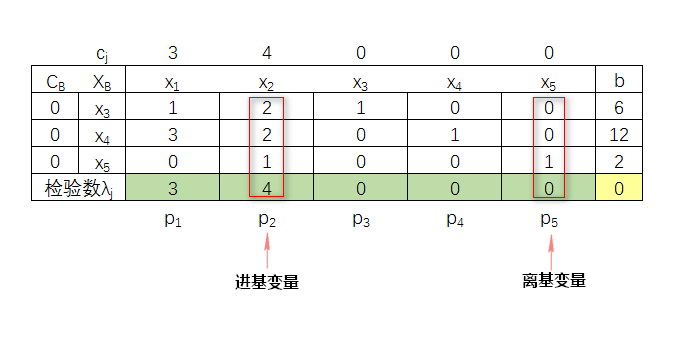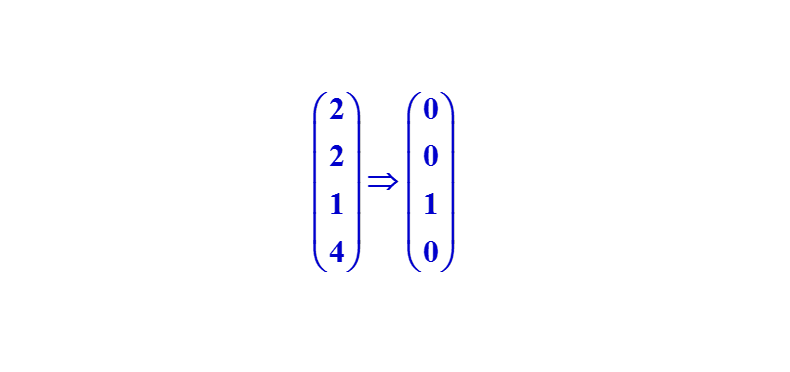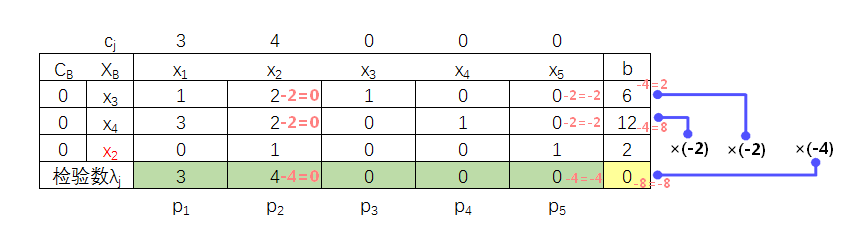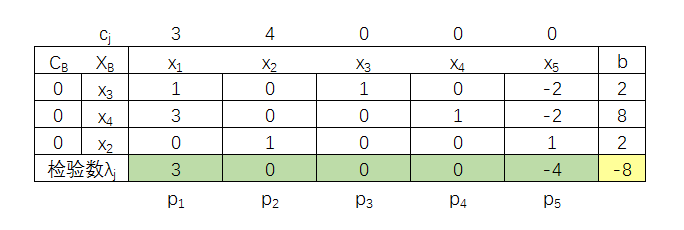X1=(0,2,2,8,0)T→Z1=8↑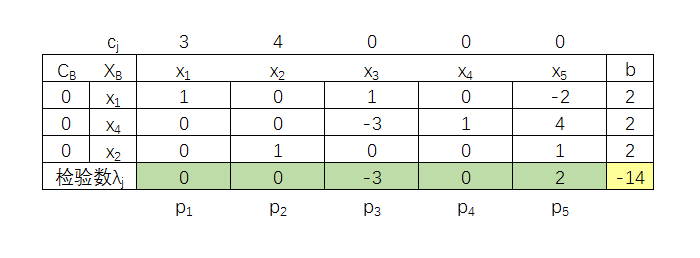X2=(2,2,0,2,0)T→Z2=14↑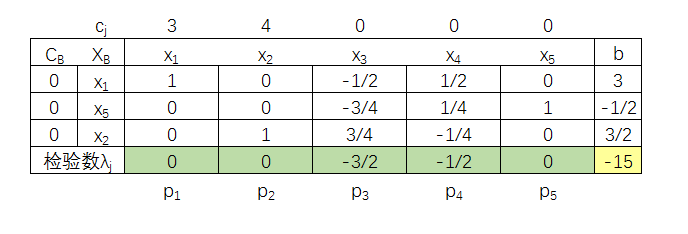X=(3,3/2,0,0,1/2)T→Z=15↑

• X0=(0,0,6,12,2)T→Z0=0
• X1=(0,2,2,8,0)T→Z1=8↑
• X2=(2,2,0,2,0)T→Z2=14↑
• X=(3,3/2,0,0,1/2)T→Z=15↑